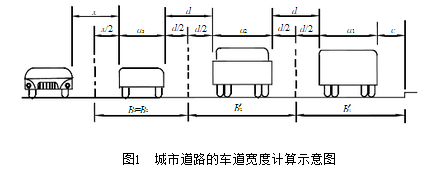# 目前车道宽度取值的依据

## 目前车道宽度取值的依据

2016-11-02 作者: xuzhiping 浏览: 4444 次

《交通工程手册》中规定：城市道路上供各种车辆行驶的路面部分，统称为机动车道，在道路上提供每一纵列车辆安全行驶的地带，称为一个车道。它的宽度决定于车辆的车身宽度，以及车辆在横向的安全距离。

（1）车身宽度  车身宽度应采用道路上经常通行的最大车辆的宽度，一般采用：大货车2.50m；大客车2.60m；小汽车2.00m。偶然通过的大型车辆，一般不作为计算的依据。

（2）横向安全距离  横向安全距离决定于车辆在行驶使的摆动、偏移的宽度，以及车身（包括装货允许的突出部分）与相邻车道或人行道侧石边缘必要的安全间隙，它同车速、路面质量、驾驶技术、交通秩序等因素有关。

$$x = 0.7+0.02 \times (v1+v2)^{3/4}$$

$$d=0.7+0.02 \times v^{3/4}$$

$$c=0.4+0.02 \times v^{3/4}$$

x——对向行车的横向安全距离；

d——同向行车的横向安全距离；

c——车辆与路缘石之间的横向安全距离；

v1、v2、v——车速。（1）靠路边的车道宽度：

$$B_1= \frac{x}{2} +a1+c$$

$$B'_1= \frac{d}{2} +a1+c$$

（2）靠中心线行驶的车道，其宽度为：

$$B_2= \frac{x}{2} +a2+ \frac{d}{2}$$

（3）同向行驶的中间车道，其宽度为：

$$B'_2= \frac{d}{2} +a2+ \frac{d}{2}$$

＜40 3.50